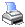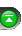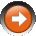﻿ Relative Strength Index (RSI)
 NinjaScript > Language Reference > Indicator Methods > Relative Strength Index (RSI)Description

Developed by J. Welles Wilder and introduced in his 1978 book, New Concepts in Technical Trading Systems, the Relative Strength Index (RSI) is an extremely useful and popular momentum oscillator. The RSI compares the magnitude of a stock's recent gains to the magnitude of its recent losses and turns that information into a number that ranges from 0 to 100.

... Courtesy of StockCharts

Syntax

 RSI(int period, int smooth) RSI(IDataSeries input, int period, int smooth)   Returns default value RSI(int period, int smooth)[int barsAgo] RSI(IDataSeries input, int period, int smooth)[int barsAgo]   Returns avg value RSI(int period, int smooth).Avg[int barsAgo] RSI(IDataSeries input, int period, int smooth).Avg[int barsAgo]

Return Value

double; Accessing this method via an index value [int barsAgo] returns the indicator value of the referenced bar.

Parameters

 input Indicator source data (?) period Number of bars used in the calculation smooth Smoothing period

Examples

 // Prints the current value of a 20 period RSI using default price type double value = RSI(20, 3); Print("The current RSI value is " + value.ToString());   // Prints the current value of a 20 period RSI using high price type double value = RSI(High, 20, 3); Print("The current RSI value is " + value.ToString());

Source Code

You can view this indicator method source code by selecting the menu Tools > Edit NinjaScript > Indicator within the NinjaTrader Control Center window.